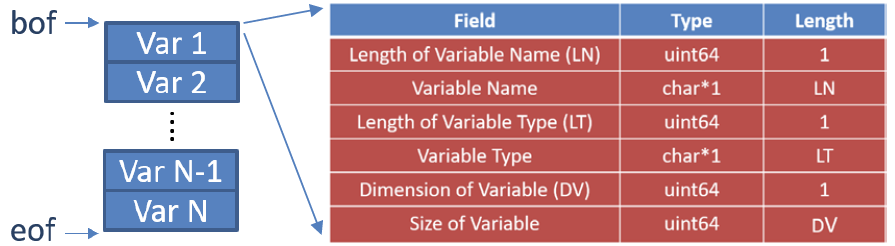# BHV2 Binary Structure

NOTE: If you just want to know how to read BHV2 in MATLAB, see "File Formats Supported by NIMH MonkeyLogic". This document is for someone who wants to develop their own BHV2 reader in different programming languages.

BHV2 is a custom binary format designed to store MATLAB variables. It has a very simple structure that can be read with a recursive algorithm.

BHV2 has no file header and just begins with variable blocks. Each variable block has 6 fields of a variable header, as shown in the following diagram. The 1st, 3rd and 5th fields indicate the lengths of the 2nd, 4th and 6th fields, respectively.If the variable type is one of the MATLAB primitive data types (char, integers, single, double, logical, etc.), then the content of the variable follows those 6 fields in column-major order. For example, if A is a 2-by-2 double matrix, the byte order of A will be:

>> A = [1 2; 3 4]

A =

1    2
3    4

 1 A 6 double 2 [2 2] 1 3 2 4

The type of the last 4 data fields above (i.e., 1, 3, 2, 4) is double, so you should read them as doubles, when you build your own reader.

If the variable type is struct, there is one more field of uint64 that indicates the number of fields in the struct, followed by the first field of the first struct array.

>> AA = [struct('a',1,'b','def') struct('a',2,'b','ghi') struct('a',9,'b','xyz')]   % 2 fields, a & b

AA =

1×3 struct array with fields:

a
b

 2 AA 6 struct 2 [1 3] 2 AA(1).a AA(1).b AA(2).a
 AA(2).b AA(3).a AA(3).b

Note that each struct field (e.g., AA(1).a) can contain any type of variables so each starts with its own variable headers (6 fields). See "Byte order of a struct" below.

If the variable type is cell, each cell of the cell array is in column-major order.

>> AAA = cell(3,2);

 3 AAA 4 cell 2 [3 2] AAA{1,1} AAA{2,1} AAA{3,1} AAA{1,2}
 AAA{2,2} AAA{3,2}

## Byte order of a struct

A(1).a = [1 2 3];
A(1).b = 'xyz';
A(2).a = [5 6; 7 8];
A(2).b = '';

 1 [1x1 uint64] % length('A') A [1x1 char] % struct name 6 [1x1 uint64] % length('struct') struct [1x6 char] % variable type 2 [1x1 uint64] % dimension of variable [1 2] [1x2 double] % size of struct 2 [1x1 uint64] % number of fields in A 1 [1x1 uint64] % length('a') a [1x1 char] % name of the first field 6 [1x1 uint64] % length('double') double [1x6 char] % variable type 2 [1x1 uint64] % dimension of variable [1 3] [1x2 double] % size of variable 1 2 3 [1x3 double] % content of the first field 1 [1x1 uint64] % length('b') b [1x1 char] % name of the second field 4 [1x1 uint64] % length('char') char [1x4 char] % variable type 2 [1x1 uint64] % dimension of variable [1 3] [1x2 double] % size of variable xyz [1x3 char] % content of the second field 1 [1x1 uint64] % length('a') a [1x1 char] % name of the first field 6 [1x1 uint64] % length('double') double [1x6 char] % variable type 2 [1x1 uint64] % dimension of variable [2 2] [1x2 double] % size of variable 5 7 6 8 [2x2 double] % content of the first field 1 [1x1 uint64] % length('b') b [1x1 char] % name of the second field 4 [1x1 uint64] % length('char') char [1x4 char] % variable type 2 [1x1 uint64] % dimension of variable [0 0] [1x2 double] % size of variable '' [0x0 char] % content of the second field (end)

The last byte in the above example does not exist since its content is blank. Note that the content of A(2).a is written as [5 7 6 8], not [5 6 7 8], since arrays are in column major order in MATLAB.

## Byte order of a cell

A = cell(2,2);
A{1 1} = [1 2 3];
A{1,2} = 'xyz';
A{2,1} = [5 6; 7 8];
A{2,2} = '';

 1 [1x1 uint64] % length('A') A [1x1 char] % cell array name 4 [1x1 uint64] % length('cell') cell [1x4 char] % variable type 2 [1x1 uint64] % dimension of variable [2 2] [1x2 double] % size of cell array 0 [1x1 uint64] % A{1,1} has no name '' [0x0 char] % no name 6 [1x1 uint64] % length('double') double [1x6 char] % variable type 2 [1x1 uint64] % dimension of variable [1 3] [1x2 double] % size of variable 1 2 3 [1x3 double] % content of A{1,1} 0 [1x1 uint64] % A{2,1} has no name '' [0x0 char] % no name 6 [1x1 uint64] % length('double') double [1x6 char] % variable type 2 [1x1 uint64] % dimension of variable [2 2] [1x2 double] % size of variable 5 7 6 8 [2x2 double] % content of A{2,1} 0 [1x1 uint64] % A{1,2} has no name '' [0x0 char] % no name 4 [1x1 uint64] % length('char') char [1x4 char] % variable type 2 [1x1 uint64] % dimension of variable [1 3] [1x2 double] % size of variable xyz [1x3 char] % content of A{1,2} 0 [1x1 uint64] % A{2,2} has no name '' [0x0 char] % no name 4 [1x1 uint64] % length('char') char [1x4 char] % variable type 2 [1x1 uint64] % dimension of variable [0 0] [1x2 double] % size of variable '' [0x0 char] % content of A{2,2} (end)

Again, the fields that have any 0-sized dimension are not written to the file. And note that not only a numeric matrix (A{2,1}) but also a cell (‘A’ itself) is arranged in column-major order. In other words, A{1,2} is placed after A{2,1} in the file.

The National Institute of Mental Health (NIMH) is part of the National Institutes of Health (NIH), a component of the U.S. Department of Health and Human Services.